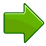# SimilarTwo shapes are Similar when one can become the other after a resize, flip, slide or turn.

## Resizing

If one shape can become another using Resizing (also called dilation, contraction, compression, enlargement or even expansion), then the shapes are Similar:

These Shapes are Similar!If there is no need to resize, then the shapes are better called Congruent*.

## There may be Turns, Flips or Slides, Too!

Sometimes it can be hard to see if two shapes are Similar, because you may also need to turn, flip or slide a shape.

Rotation## Examples

Here are 3 examples of shapes that are Similar:Resized Resized and Reflected Resized and Rotated

## Why is it Useful?

When two shapes are similar, then:

• corresponding angles are equal, and
• the lines are in proportion.

This can make life a lot easier when solving geometry puzzles, as in this example:

### Example: What is the missing length here?Notice that the red triangle has the same angles as the blue triangle ...

... they both have one right angle, and a shared angle in the left corner

In fact we can flip the red triangle over, rotate it a little, resize it, and it will fit exactly on top of the blue triangle. So they are similar triangles.

So the line lengths are in proportion:

• The blue triangle has two sides with the ratio 130/127
• The red triangle has matching sides in the ratio ?/80

and we can calculate:

### ? = 80 × 130127 = 81.9

(No fancy calculations, just common sense.)

## Congruent or Similar?

Shapes are Congruent when they are the same size (but may have been rotated, reflected or moved). So when the shapes become the same:

When we ...   Then the shapes are ...
... only Rotate, Reflect and/or Translate### Congruent

... also need to Resize## *Are Congruent Shapes also Similar?

Most people (including us) agree that "Congruent shapes are also Similar".

### Example:We can move and rotate the orange shape to exactly match the blue shape, so the two shapes are Congruent.

We don't have to resize for the shapes to be similar! So they are also Similar even though no resizing was needed.

781, 3272, 6050, 6051, 6052, 3273, 3274, 3275, 631, 632, 1802, 1803